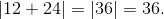# High School Math : High School Math

## Example Questions

### Example Question #81 : High School Math

Solve the expression below.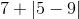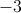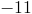Explanation: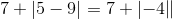For an absolute value, the term inside the bars will always become positive.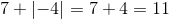### Example Question #82 : High School Math

Solve the expression below.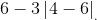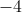Explanation: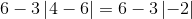For an absolute value, the term inside the bars will always become positive.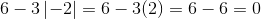### Example Question #83 : High School Math

Solve the absolute value expression.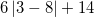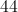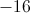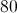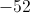Explanation:Simplify the absolute value, as if it were a parenthesis.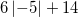The absolute value ofis. Remember that absolute values are always positive.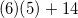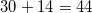### Example Question #84 : High School Math

Is the inequality below true or false?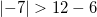False; the sign should be changed to "less than"

False; the two terms are equal

True

True

Explanation:

An absolute value is always the positive solution.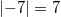We can compare the terms by substituting the positive value of, and solving the right side of the inequality.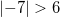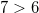The inequality is a true expression.

### Example Question #85 : High School Math

Evaluate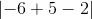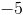Explanation:

To solve problems with absolute value, first solve inside the absolute value signs.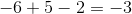.

The absolute value ofisbecause the negative sign is inside the absolute value signs. Remember the absolute value is the distance from that number to zero on the number line. Therefore, the absolute value is always positive.

### Example Question #86 : High School Math

Evaluate the expression.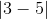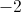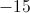Explanation:

First, treat the absolute value as a parenthesis, and evaluate the term inside.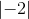The absolute value of any term is its distance from zero. A distance cannot be negative, thus, any negative term in an absolute value will be converted to a positive term.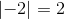### Example Question #87 : High School Math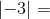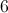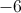Explanation:

The absolute value of a number can be thought of as its distance from zero.is three units away from zero. Therefore,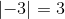.

### Example Question #88 : High School Math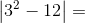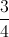Explanation:

The absolute value of a number can be thought of as its distance from zero.

We start by solving the expression inside the absolute value signs and then measure how far that is from zero.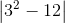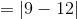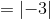is three units away from zero. Therefore,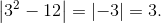### Example Question #89 : High School Math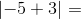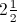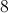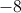Explanation:

The absolute value of a number can be thought of as its distance from zero.

We start by solving the expression inside the absolute value signs and then measure how far that is from zero.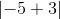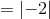is two units away from zero. Therefore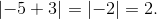### Example Question #90 : High School Math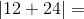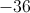Explanation:

The absolute value of a number can be thought of as its distance from zero.

We start by solving the expression inside the absolute value signs and then measure how far that is from zero.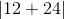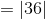isunits away from zero. That means that